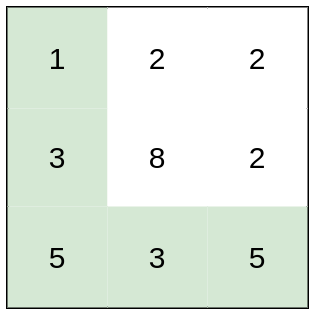# 5548. 最小体力消耗路径1.BFS,记忆化

2.二分答案，用答案对图做剪枝条件，10**7


class Solution:
def minimumEffortPath(self, heights: List[List[int]]) -> int:
que=deque([[0,0,0]])
t=[[float('inf')]*len(i) for i in heights]
t=0
while que:
i,j,k=que.popleft()
if k<=t[i][j]:
for x,y in (i+1,j),(i-1,j),(i,j-1),(i,j+1):
if 0<=x<len(heights) and 0<=y<len(heights):
z=max(k,abs(heights[x][y]-heights[i][j]))
if z<t[x][y]:
t[x][y]=z
que.append([x,y,z])
return t[-1][-1]

class Solution:
def minimumEffortPath(self, heights: List[List[int]]) -> int:
m=len(heights)
n=len(heights)
l=0
r=10**6
def dfs(i,j):
if i==m-1 and j==n-1:
return True
for x,y in [(i+1,j),(i-1,j),(i,j+1),(i,j-1)]:
if 0<=x<m and 0<=y<n and (x,y) not in visited and abs(heights[x][y]-heights[i][j])<=mid:
if dfs(x,y):return True
return False

while l<r:
mid=(l+r)//2
visited=set([(0,0)])
if dfs(0,0):
r=mid
else:
l=mid+1
return l

10-25243
09-13144710-2554
08-061万+
08-14726
01-2276
10-25147
10-2648
08-1937
09-1523